Next:7.3 Warshall's AlgorithmUp:7.2 Shortest Paths ProblemPrevious:7.2.2 Dynamic Programming Algorithm

## 7.2.3 All Pairs Shortest Paths Problem: Floyd's Algorithm

REF.
Robert W Floyd. Algorithm 97 (Shortest Path). Communications of the ACM, Volume 5, Number 6, pp. 345, 1962.
 tex2html_deferredGiven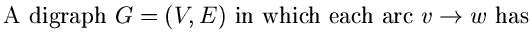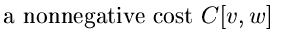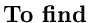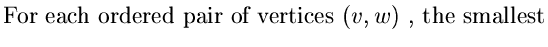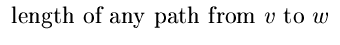Floyd's Algorithm

• This is an O(n3) algorithm, where n is the number of vertices in the digraph.
• Uses the principle of Dynamic Programming
• Let V = {1, 2,..., n}. The algorithm uses a matrix A[1..n][1..n] to compute the lengths of the shortest paths.
• Initially,
•  A[i, j] = C[i, j]  if  i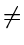j (7.12) = 0          if  i = j

Note that C[i, j] is taken as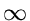if there is no directed arc from ito j.

• The algorithm makes n passes over A. Let A0, A1,..., Anbe the values of A on the n passes, with A0 being the initial value. Just after the k-1th iteration,
•  Ak - 1[i, j] =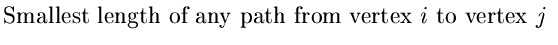(7.13)(7.14)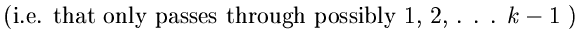See Figure 7.8

• The kth pass explores whether the vertex k lies on an optimal path from i to j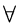i, j

•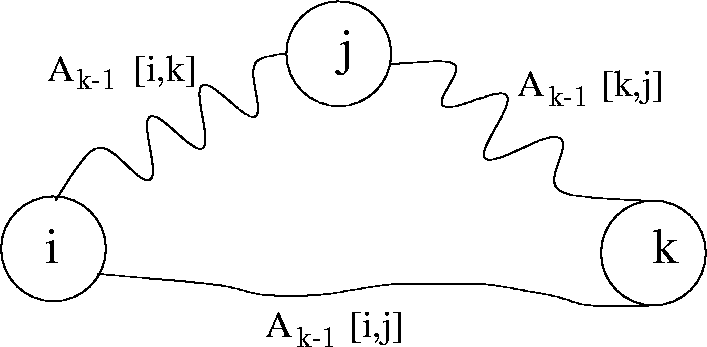• We use
• Ak[i, j] = min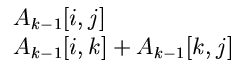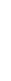• The algorithm:

• void Floyd (floatC[n - 1][n - 1], A[n - 1][n - 1])

{ int i, j, k;

{ for(i = 0, i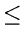n - 1;i + +)

for(j = 0;jn - 1, j + +)

A[i, j] = C[i, j];

for(i = 0;in - 1;i + +)

A[i, i] = 0;

for(k = 0;kn - 1;k + +);

{

for(i = 0;in - 1;i + +)

{

for(j = 0;jn - 1, j + +)

if(A[i, k] + A[k, j] < A[i, j])

A[i, j] = A[i, k] + A[k, j]

}

}

}

}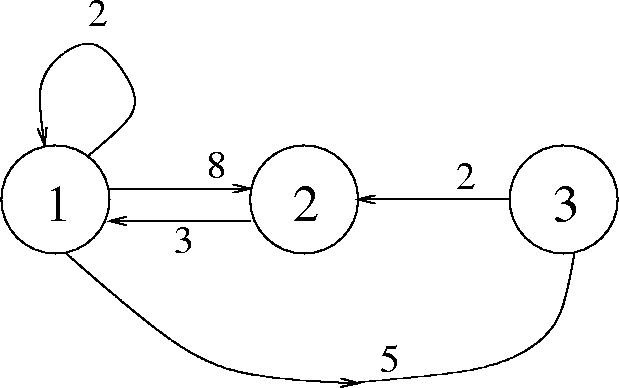Example: See Figure 7.9.

C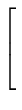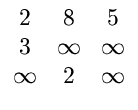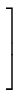A0 =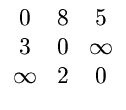;   A1 =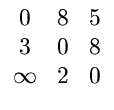(7.15) A2 =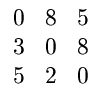;   A3 =• With adjacency matrix representation, Floyd's algorithm has a worst case complexity of O(n3) where n is the number of vertices
• If Dijkstra's algorithm is used for the same purpose, then with an adjacency list representation, the worst case complexity will be O(nelog n). Thus if e is O(n2), then the complexity will be O(n3log n) while if e is O(n), then the complexity is O(n2log n).Next:7.3 Warshall's AlgorithmUp:7.2 Shortest Paths ProblemPrevious:7.2.2 Dynamic Programming Algorithm
eEL,CSA_Dept,IISc,Bangalore